Function Repository Resource:

# LogSumExpLayer

Neural network layer that implements the LogSumExp operation on any level

Contributed by: Sjoerd Smit
 ResourceFunction["LogSumExpLayer"][] creates a NetGraph that computes the LogSumExp of an array on level 1. ResourceFunction["LogSumExpLayer"][{n1,n2,…}] computes the LogSumExp of an array on levels n1,n2,…. ResourceFunction["LogSumExpLayer"][All] creates a NetGraph that computes the LogSumExp over all values, returning a real number.

## Details and Options

The LogSumExp of a list list is equivalent to Log[Total[Exp[list]]], but the implementation used by ResourceFunction["LogSumExpLayer"] first subtracts the max from the list to prevent the Exp operator from causing overflows and/or underflows. The max is added back again at the end.
The level specifications in ResourceFunction["LogSumExpLayer"] work the same as in AggregationLayer. However, because of the internal use of ReplicateLayer, it is not possible to use "Input" "Varying" with ResourceFunction["LogSumExpLayer"].
The LogSumExp operation is very useful when dealing with very large or very small positive numbers that cannot be represented in machine precision. In that case, it is more convenient to work in log-space to make the numbers more manageable. In log-space, multiplication becomes addition, and addition is replaced with the LogSumExp operation.
ResourceFunction["LogSumExpLayer"][All] uses FlattenLayer to flatten all dimensions before aggregating.

## Examples

### Basic Examples (1)

LogSumExpLayer creates a NetGraph:

 In:=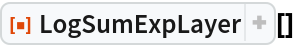Out=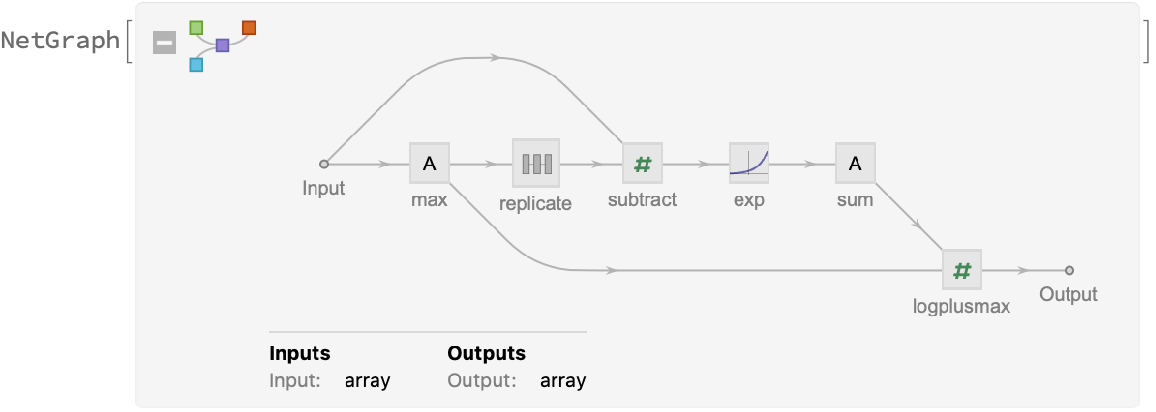### Scope (1)

Aggregate on different levels:

 In:=Out=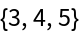In:=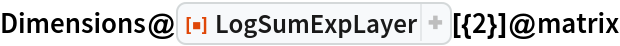Out=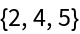In:=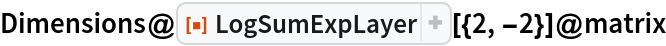Out=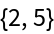In:=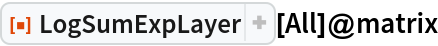Out=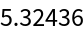### Options (4)

#### Aggregator (2)

Compute the mean instead of the sum:

 In:=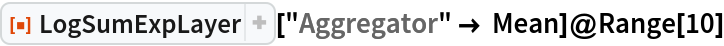Out=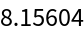Compare with the ordinary method of evaluation:

 In:=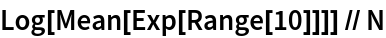Out=#### LevelSorting (2)

Using this option should not be necessary under normal circumstances. It exists mainly for cases where the default sorting does not work correctly. By default, LogSumExpLayer sorts the level specifications in such a way that the ReplicateLayer sequence in the NetGraph will reshape the computed maxima correctly:

 In:=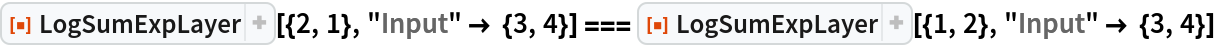Out=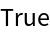Without the correct sorting order, the network cannot be constructed:

 In:=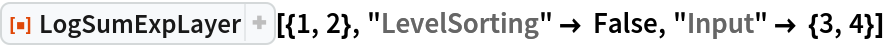Out=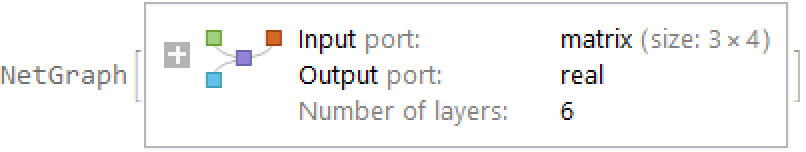In:=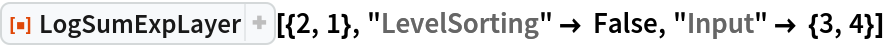Out=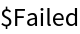### Properties and Relations (5)

Calculate the LogSumExp of a list:

 In:=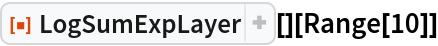Out=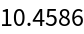This is equivalent to chaining the functions Exp, Total and Log together:

 In:=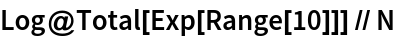Out=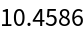However, for very small or very large numbers, machine precision numbers will overflow or underflow during the computation:

 In:=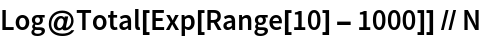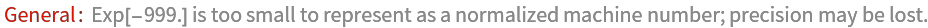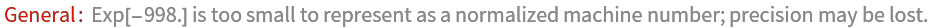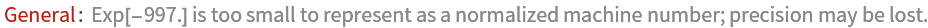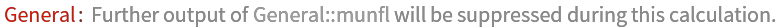Out=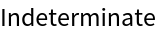You need arbitrary precision numbers for this operation:

 In:=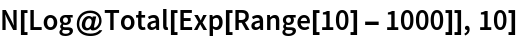Out=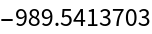LogSumExpLayer will still be able to work in machine precision for such numbers:

 In:=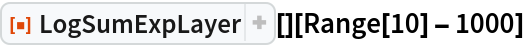Out=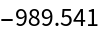In:=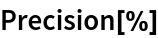Out=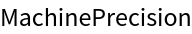### Possible Issues (1)

Positive and negative level specs can only be used together as long as they remain ordered:

 In:=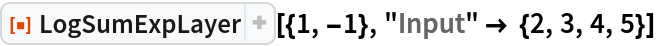Out=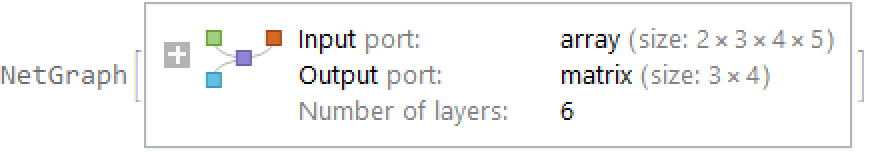In:=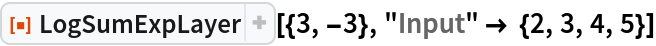Out=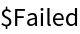Sjoerd Smit

## Version History

• 2.0.0 – 20 September 2019
• 1.0.0 – 30 July 2019